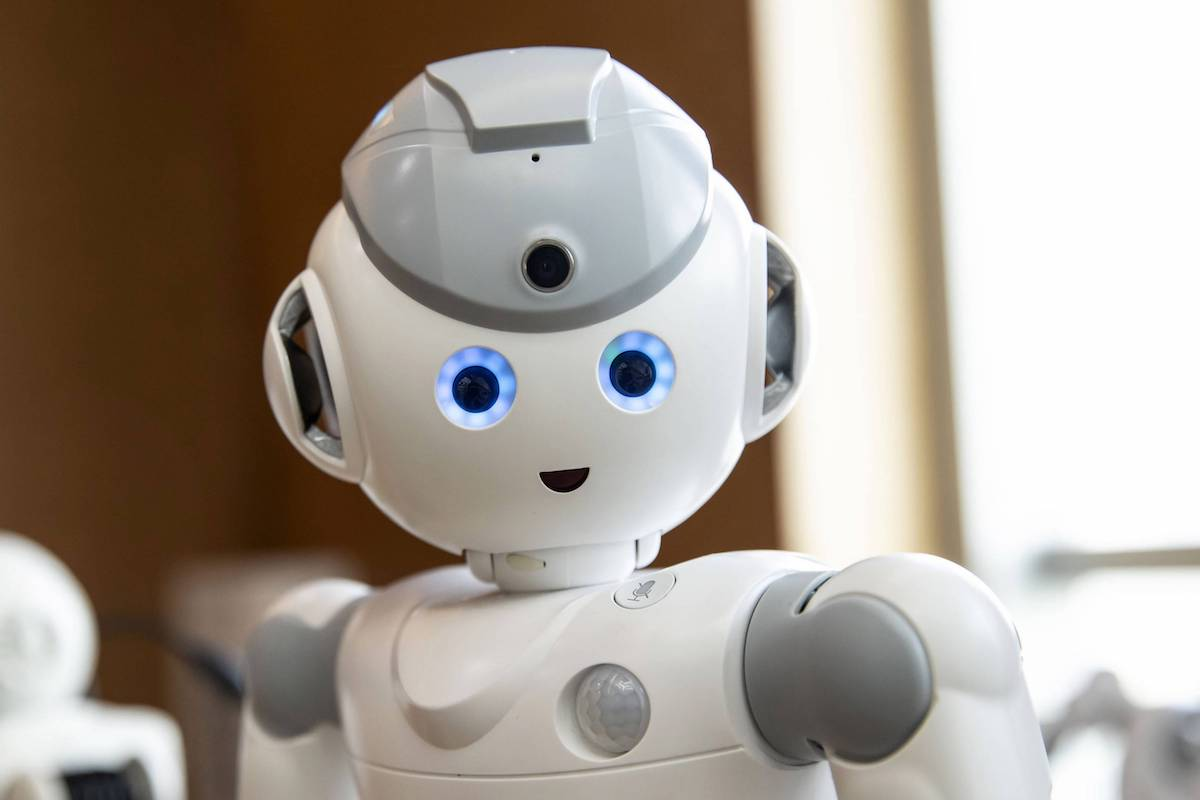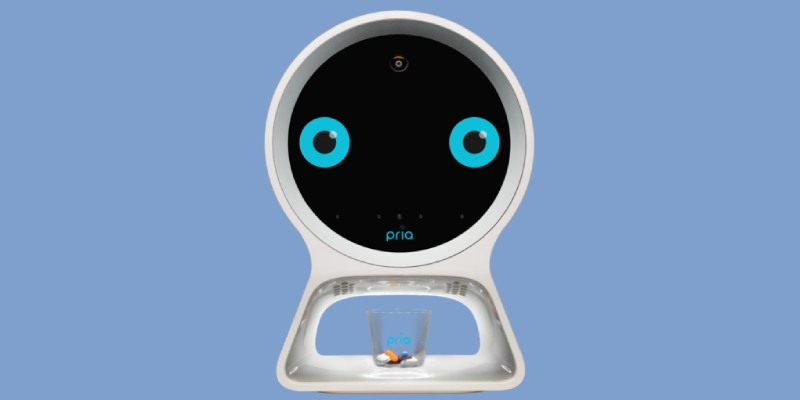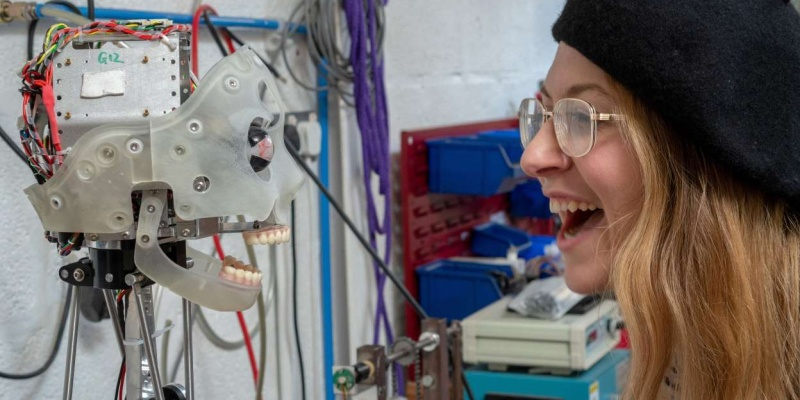Here are the coolest robots of 2019 so far

While innovation is slowing in some tech niches, robotics is moving forward at speed. From health helpers to android artists, here’s our look at the coolest robots we have seen thus far in 2019.• How can robots actually help me? Most consumer robots focus on health, education and companionship. However, some bots can fold your clothes and replace your presence in the office!
• How smart are robots in 2019? Some are extremely intelligent. Ai-Da can draw your portrait, for instance.
• What are the most useful robots right now? Check out the cleaning ‘bots in our Robots collection.

It was back in the 1950s that intelligent, human-like robots first starting making an appearance in sci-fi movies. Ever since, reality has been trying to catch up — but the gap is closing fast. Here are the coolest robots we’ve seen this year.

Stanley Black & Decker Pria

Black & Decker is best known for creating DIY tools, but the company has teamed up with Stanley healthcare to create something very different.

Pria has been designed to assist elderly folks with their medications. This helpful ‘bot helps you weigh out the right dosage, before giving timely reminders throughout the day. In addition, Pria can also be used for video-calling friends and family.Pria offers timely health reminders

Sharp RoBoHon 2nd-Generation Toy Robot

Just as with Sony’s famous Aibo robot dog, the primary function of RoBoHon is companionship. While the original version was only available in Japan, version two is getting a wider release.

The robot has many of the same features as a smartphone, including Wi-Fi and LTE connectivity. It can also walk around, respond to your voice, dance to music, take photos, and even control smart home appliances.

Peeqo Intelligent Gif Robot

Many robots can now hold a vaguely intelligent conversation. But why talk when you can gif? That’s the concept behind Peeqo, a tabletop robot that originally started life as a viral DIY project. Powered by a Raspberry Pi, this quirky device responds with videos to whatever you say, without ever using the same clip twice.

The kit version of this robot is currently seeking crowdfunding on Kickstarter; the first units are set to ship in September.

UBTech Walker Humanoid Robot

We first encountered Walker at CES 2018. A year later, this humanoid bot is looking a lot more human. Weighing in at a muscular 170 pounds, Walker has SLAM (Simultaneous Localization and Mapping) technology on board along with 36 actuators.

Put together, these components allow the robot to walk around, avoid obstacles and lift objects of up to 11 pounds (5 kg).

Sony Aibo Robot Dog

Ah, Aibo. This cute canine-like machine mimics the wagging tail and bright eyes of man’s best friend. For anyone without the space or time to raise a puppy, this is the next best thing.

In addition, Aibo is smart. The robot learns about its environment through observation. Like a real pet, it even comes to recognize the whole family and understand the relationships between the humans. Such a good bot.

Samsung Bot Air Filter Robot

Among the many robots launched by Samsung at CES 2019, the Bot Air stands out as the most innovative. It might look like a rolling waste paper basket, but this robot scoops up another kind of trash: airborne particles.

In a home environment, Bot Air will roll from room to room, monitoring the air quality. Where it finds pollutants or allergens, it stays for a while and cleans the air. For folks who live in urban environments, it could be a huge health upgrade.

Ai-Da Artistic Robot

There’s little chance that anyone other than British gallery owner, Aidan Meller, will ever own an Ai-Da. But this incredible robot still merits a place on this list, thanks to its artistic talent.

Ai-Da can draw a perfect portrait of any face on sight, without the need for human intervention. The robot will eventually be fitted with human-like anatomy, and will use AI to mimic facial expressions.Ai-Da has serious artistic talent (Credit: Reuters/Matthew Stock)

Sphero RVR Hackable Robot

You probably know Sphero for making those RC balls. While those devices are very much like toy cars, the RVR is something completely different. Currently seeking crowdfunding on Kickstarter, this four-wheeler allows owners to get creative with code and components. That’s because the RVR is essentially a rolling Raspberry Pi.

The robot actually comes with an impressive list of parts, but owners will be able to add other accessories for unique projects.

Coolest robots of 2019

Which of these robots would you take home? Share your perfect robotic set-up in the comments!

The Gadget Flow Daily Digest highlights and explores the latest in tech trends to keep you informed. Want it straight to your inbox? Subscribe ➜

Meet Mark Myerson

Mark is best known for writing about apps, but he also loves the tactile, hardware side of technology. Being a professional photographer, he's pretty handy with a camera, and he's a self-confessed tweetaholic.

2
Click here to tag users that participate in this comment thread.
Click or drag your image here (Maximum Size 4MB, Accepted formats JPG, PNG, GIF).
• 😂
• ❤️
• ♥️
• 😍
• 😭
• 😊
• 😒
• 😘
• 💕
• ☺️
• 😩
• 👌
• 😔
• 😏
• 😁
• 😉
• 👍
• 🙏
• 😌
• 🎶
• 😳
• 🙌
• 🙈
• 😢
• 😎
• ✌️
• 👀
• 😅
• 😴
• 😄
• 💜
• 💔
• 💯
• 😑
• 💖
• 💙
• 😕
• 💁
• 😜
• 😞
• 😋
• 😐
• 😪
• 👏
• 💘
• 💗
• 💞
• ⬅️
• 🙊
• 💋
• 👉
• 🌸
• 😱
• 🔥
• 😡
• 😃
• 🎉
• 👊
• 😫
• 📷
• 🌹
• 😝
• 💪
• 💀
• ☀️
• 💛
• 😤
• 🌚
• 😆
• 😓
• 👈
• ✔️
• 😻
• 😀
• 😷
• 💚
• 👋
• 😣
• 💓
• ▶️
• ◀️
• ↪️
• ↩️
• 👑
• 😚
• 😛
• 😥
• 😇
• 🎧
• 😖
• 😠
• 😬
• 🌟
• 🔫
• 🙋
• 👎
• 💃
• 🎵
• 😶
• 💫
• 👇
• 🔴
• 🙅
• 💥
• 💭
• 👅
• 💩
• 😰
• 💎
• 🙆
• 🍕
• 😹
• 🌞
• 🍃
• 💦
• 🐧
• 💤
• 🚶
• ✈️
• 🎈
• 🎀
• ☑️
• 😟
• 🔞
• 😨
• 🍀
• 🌺
• 🎤
• 👐
• 👻
• 🌴
• ‼️
• 💅
• 👽
• 🙇
• 👼
• 👯
• ❄️
• ☝️
• 😙
• 🌈
• 🌙
• 💟
• 💝
• 🎁
• 🍻
• 😧
• 🌍
• 🎥
• ♣️
• ✖️
• 🏃
• 🌻
• 🌎
• 💐
• 🐶
• 💰
• 🌿
• 👫
• 🍂
• 🌷
• 🎂
• 🐱
• 😵
• 👆
• 😮
• 😯
• 🏀
• 🎄
• 💍
• 🌝
• 😲
• 👭
• 💸
• 😿
• 🙉
• 💨
• 🌵
• ♨️
• ☎️
• 🍁
• 👸
• 💆
• 💌
• 🏆
• 🙍
• 🎊
• 🌼
• 🔪
• 👄
• 🍟
• 🍩
• 😦
• 🌊
• 💣
• 🆗
• 🌀
• 🚀
• 💏
• 🍭
• 🎬
• 🐷
• 😈
• 👿
• 🐝
• 😽
• 💢
• 🎼
• 🎅
• 🌏
• 🏈
• 🎸
• ♦️
• 🐼
• 💬
• 🍓
• 😼
• 🍌
• 🍉
• 😸
• ♠️
• 🔝
• 🍆
• 🔮
• 🍴
• 📲
• 📱
• ⚠️
• 🙀
• 🔸
• 👶
• 🐾
• 👣
• 🍺
• 🍷
• 📹
• 🐰
• 🍹
• 🚬
• 👾
• 🍑
• 🐍
• 🐢
• 🍒
• 😗
• 🐸
• 🌌
• 🚨
• 🐣
• 📕
• 🍬
• 🍔
• 🐻
• 🐯
• 🚗
• 🍦
• 🍍
• 🌾
• 💉
• 🚮
• 🍫
• ▪️
• 📺
• 💊
• 🐙
• 🎃
• 🍇
• 😺
• 💿
• 🍸
• 🍰
• 🎮
• ™️
• ⬇️
• 🚫
• 💄
• 🐳
• 📝
• ®️
• 🍪
• 🐬
• 🔊
• 👨
• 🐥
• 🐒
• 📚
• 👹
• 💂
• 📢
• ✂️
• 👧
• 🎓
• ⚾️
• 🚦
• 👩
• 🎆
• 🌠
• 🆘
• 🍄
• 😾
• 🛅
• 👠
• 🎯
• 🏊
• 🔑
• 👙
• 👪
• 🐘
• 💧
• 🌱
• 🍎
• 🆒
• 📞
• 💵
• 🏡
• 📖
• 💇
• 💻
• 💡
• 🔙
• 👦
• 🔐
• 🙎
• 🍊
• ↔️
• 🌅
• 🍗
• 🔵
• 🚘
• 🍧
• 🐦
• 🌛
• 👓
• 🐐
• 🌃
• 👵
• 🌑
• 👬
• 🛃
• 🐠
• 🏠
• 🔃
• 🌜
• 📍
• 🌕
• 👟
• 🍋
• 🍼
• 🎨
• ✉️
• 🍝
• 🎐
• 🍥
• 🌲
• 🆙
• ⬆️
• ↗️
• ↘️
• ↙️
• 🎭
• 👃
• 🐽
• 🐟
• 👳
• 🐨
• 👂
• ✳️
• 🔹
• 🚿
• 🐛
• 🍜
• 🎩
• 👰
• 🏁
• 🐴
• 🐵
• 🚼
• 🆕
• 🆓
• 🎇
• 🌽
• 🎾
• 🔋
• 🐺
• 🗿
• 🐮
• 📣
• 👴
• 👗
• 🔗
• 🐔
• 🍳
• 🐋
• 🌳
• 🍱
• 📌
• 🔜
• 🔁
• 🐉
• 🐹
• 🏄
• 🐭
• 🌒
• 🚙
• 🅰️
• ⁉️
• 🔌
• 🌓
• 🔱
• 🍞
• 👮
• 🍵
• 🎣
• 🌔
• 🚲
• 👤
• 🍚
• 📻
• 🐤
• ⤵️
• 🌘
• 🌗
• 🔘
• 🐑
• 👱
• 🌖
• 🔒
• 🍏
• 👺
• 🚩
• 🔄
• 🐎
• 🍤
• 🌄
• 🌋
• 🐓
• 📥
• 💒
• 🍣
• 🍨
• 🍅
• 🐇
• ✴️
• 🔺
• 🔆
• 👲
• 🏪
• 👥
• 🐞
• 🔻
• ⤴️
• 📛
• 🛀
• 🐊
• 🌰
• 🐕
• 🐈
• 🔨
• 🍖
• 🐚
• ❇️
• 🅱️
• Ⓜ️
• 🐩
• 🍲
• 👖
• 🍯
• 🎹
• 🔓
• 🗽
• 💲
• 🏂
• 💮
• 👔
• 💠
• 🚺
• 🐜
• 🌇
• 🅾️
• 🐲
• 🐌
• 📀
• 👕
• 🎲
• 🎎
• 🎱
• 🚌
• 🍮
• 🎌
• 〽️
• 🐫
• 🍛
• 🚂
• 🏥
• 🔷
• 🎋
• 🔔
• 🍐
• 🔶
• 🌐
• 🚪
• 🕕
• 🚔
• 📩
• 🌂
• 🎷
• 🚴
• 🍡
• 🏢
• 🚣
• 👒
• 👞
• 🏩
• 🗻
• 🐪
• 👜
• 🎺
• 🏫
• 🐄
• 🌆
• 👷
• 🚽
• 🐖
• 🔰
• 🎻
• 🔛
• 💳
• 🆔
• 🎡
• 🎳
• 💈
• 👛
• 🎢
• 🐀
• 📅
• 🏉
• 🐏
• 🔼
• 🔲
• 📴
• 🗼
• 👘
• 🚢
• 🔎
• 🔍
• 🚒
• 🕦
• 🚓
• 🃏
• 🌉
• 📦
• 🚖
• 📆
• 🏇
• 🐅
• 👢
• 🚑
• 🔳
• 🐗
• 🎒
• 💷
• 🐂
• 🍙
• 🆚
• 🔚
• 🅿️
• 👡
• 💺
• 🚕
• 💼
• 📰
• 🎪
• 🔯
• 🚹
• 🏰
• 🔦
• 🌁
• 🎍
• 🎫
• 🚁
• 💽
• 🚍
• 🍈
• 🏤
• 🔟
• 📓
• 🔕
• 🍢
• 🎏
• 🎠
• 🐡
• 📈
• 🍠
• 🎿
• 🕛
• 📶
• 🚧
• #
• 📡
• 💶
• 👚
• 📒
• 🐆
• 🔅
• 🕒
• 🏬
• 🚚
• 🍶
• 🚃
• 🚤
• 📼
• 🕐
• 🐃
• 🔽
• 💴
• 🔇
• 🎽
• 🕑
• 📎
• 🏧
• 🎦
• 🔭
• 🎑
• 📘
• ◻️
• 📮
• 📧
• 🐁
• 🚄
• 🔩
• 🆖
• 🏨
• 🚾
• 🏮
• 🔂
• 📬
• 📉
• 📗
• 🚜
• 🚇
• 📋
• 📵
• 🕓
• 🚭
• 🎰
• 🕔
• 🛁
• 📜
• 🚉
• 🍘
• 🏦
• 🔧
• 🈯️
• 🚛
• 📄
• 📊
• 🚷
• 📳
• 🕙
• 🕘
• 🚅
• 🚐
• 🚊
• 🕗
• 🈳
• 🚥
• 🚵
• 🔬
• 🏯
• 🔖
• 📑
• 👝
• 🆎
• 📃
• 🎴
• 🕚
• 📠
• 🕖
• 💱
• 🔉
• 💹
• 🆑
• 💾
• 🏣
• 🔈
• 🗾
• 🈺
• 🀄
• 📨
• 📙
• 🚻
• 🈚️
• 🈶
• 📐
• 🚋
• 🈸
• 🚎
• 🈷
• 🔢
• 📔
• 🈲
• 🈵
• 📯
• 🏭
• 🚸
• 🚆
• 📏
• 📟
• 🉑
• 🈴
• 🔏
• 🕜
• 🈂️
• 📤
• 🔀
• 📫
• 🚈
• 🕤
• 🚏
• 📂
• 📁
• 🚰
• 📇
• 🕝
• 🚝
• 🕧
• 🕥
• 🔤
• 📪
• 🕟
• 🚞
• 🚯
• 🕞
• 🕢
• 🕠
• 🔠
• 📭
• 🔣
• 🚡
• 🕣
• 🕡
• 🔡
• 🚠
• 🈁
• 🛂
• 🚱
• 🚟
• 🛄
• 🚳
• 🏳‍🌈
• 🕵
• 🤗
• 🤖
• 🤕
• 🤔
• 🤓
• 🤒
• 🤑
• 🤐
• 🙄
• 🙃
• 🙂
• 🙁
• 🤘
• 🖖
• 🖕
• 🖐
• 🕶
• 👁‍🗨
• 👁
• 🏋
• 🕴
• 🏌
• ❣️
• 🛢
• 🛡
• 🛠
• 🛏
• 🛎
• 🛍
• 🛌
• 🛋
• 🗳
• 🗡
• 🗞
• 🗝
• 🗜
• 🗓
• 🗒
• 🗑
• 🗄
• 🗃
• 🗂
• 🖼
• 🖲
• 🖱
• 🖨
• 🖥
• 🖍
• 🖌
• 🖋
• 🖊
• 🖇
• 🕹
• 🕳
• 🕰
• 🕯
• 📿
• 📽
• 📸
• 🏺
• 🏷
• 🏴
• 🏳
• 🎞
• 🎛
• 🎚
• 🎙
• 🌡
• 🛳
• 🛰
• 🛬
• 🛫
• 🛩
• 🛥
• 🛤
• 🛣
• 🗺
• 🕍
• 🕌
• 🕋
• 🏟
• 🏞
• 🏝
• 🏜
• 🏛
• 🏚
• 🏙
• 🏘
• 🏗
• 🏖
• 🏕
• 🏔
• 🏎
• 🏍
• 🏹
• 🏸
• 🏵
• 🏓
• 🏒
• 🏑
• 🏐
• 🏏
• 🏅
• 🎟
• 🎗
• 🎖
• 🧀
• 🍿
• 🍾
• 🍽
• 🌶
• 🌯
• 🌮
• 🌭
• ☃️
• ☂️
• 🦄
• 🦃
• 🦂
• 🦁
• 🦀
• 🕸
• 🕷
• 🕊
• 🐿
• 🌬
• 🌫
• 🌪
• 🌩
• 🌨
• 🌧
• 🌦
• 🌥
• 🌤
• 🗣
• 🖤
• 🗨
• 🥚
• 🛑
• 🥁